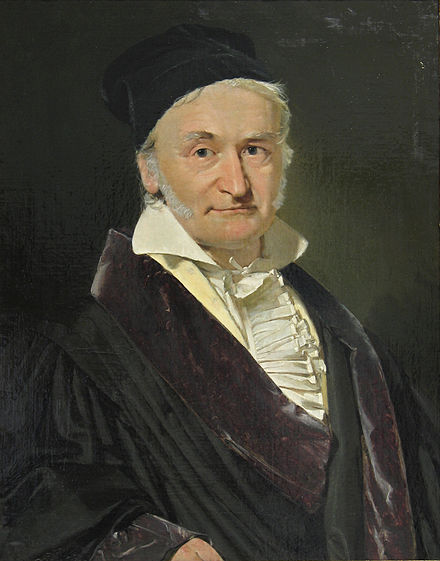Sum of Numbers¶Surely, you have heard this man’s name: Carl Friedrich Gauss. He grew up to be a famous mathematician. In fact, his influence on the world of mathematics was quite amazing.

Note

Get out of your box sometime, put your keyboard aside, and read some of the papers written by these folks. My favorite book on this is “God Created the Integers” [Haw07] edited by Stephen Hawking. It is a tough read, but amazing to see how these thinkers came up with discoveries we use even today! This book also has papers by George Boole, and Alan Turing.

Busy Work!¶

The story goes that Gauss was irritating his math teacher, way back in 1700, and that teacher assigned a problem for Gauss to solve that would keep him busy.

Find the sum of all the numbers from 1 to 100

Gauss, being no dummy, took this problem on and immediately found a pattern. Patterns help us generalize a problem and come up with a simple way to find the answer.

In this case, Gauss found a pattern this way.

• Split the list into two parts
• Reverse the second list
• Add up the two lists
• Sum the two lists

Here is an example.: Sum up all the numbers from 1 to 100

1   2   3   4 ...  47  48  49  50
100  99  98  97 ...  54  53  52  51
-----------------------------------
101 101 101 101 ... 101 101 101 101

There are 50 terms in the final result, so the answer is 50*101 = 5050. Furthermore, he came up with this general formula for the result:Suppose we want the formula for the sum of numbers between any two numbers. We can use Gauss’s formula to find the answer:We will use this formula in our testing later.

I Hate Math¶

That is why we use computers, to do our busy work.

Note

Actually, I like math, and I wish I had studied it more in school. It is never too late to learn more. Go read that book!

Lets build a program to do Gauss’s busy work for him. This should be easy:

#include <iostream>

int main( void ) {
std::cout << "Gauss's Busy Work (v1)" << std::endl;

int sum = 0;
int number = 100;

for(int i=1; i<=number; i++) sum += i;
std::cout << "The sum of all nubers from 1 to " << number;
std::cout << " is " << sum << std::endl;
}

Simple enough. We are going to use this code as a basis for exploring how memory is really managed in a modern computer.

First, let’s make this code more complex. We will load each number into an array, then sum up that array:

#include <iostream>

const int NUMBER = 100;
int numbers[NUMBER];

int main( void ) {
std::cout << "Gauss's Busy Work (v2)" << std::endl;

int sum = 0;
int number = NUMBER;

// load the array
for(int i=0; i < number; i++) numbers[i] = i+1;

for(int i=0; i<number; i++) sum += numbers[i];

std::cout << "The sum of all nubers from 1 to " << number;
std::cout << " is " << sum << std::endl;
}

To make it even more complex, let’s rework this so it uses a two dimensional array!

Note

I said this was busy work!

#include <iostream>

const int SIZE = 10;
int numbers[SIZE][SIZE];

int main( void ) {
std::cout << "Gauss's Busy Work (v3)" << std::endl;

int sum = 0;
int nrows = SIZE;
int ncols = SIZE;
int number = nrows * ncols;

// load the array
int i = 1;
for(int r = 0; r < nrows; r++)
for(int c = 0; c < ncols; c++)
numbers[r][c] = i++;

// sum the numbers
for(int r = 0; r < nrows; r++)
for(int c = 0; c < ncols; c++)
sum += numbers[r][c];

std::cout << "The sum of all nubers from 1 to " << number;
std::cout << " is " << sum << std::endl;
}

And here is the final version. It has one subtle change:

#include <iostream>

const int SIZE = 10;
int numbers[SIZE][SIZE];

int main( void ) {
std::cout << "Gauss's Busy Work (v4)" << std::endl;

int sum = 0;
int nrows = SIZE;
int ncols = SIZE;
int number = nrows * ncols;

// load the array
int i = 1;
for(int r = 0; r < nrows; r++)
for(int c = 0; c < ncols; c++)
numbers[r][c] = i++;

// sum the numbers
for(int c = 0; c < ncols; c++)
for(int r = 0; r < nrows; r++)
sum += numbers[r][c];

std::cout << "The sum of all numbers from 1 to " << number;
std::cout << " is " << sum << std::endl;
}

Just for completeness, here is a Makefile you can use to build all of these programs.

SRCS	:= $(wildcard *.cpp) OBJS :=$(SRCS:.cpp=.o)
TGTS	:= $(SRCS:.cpp=) all:$(TGTS)

%.:	%.o
g++ -o $@$<

%.o:	%.cpp
g++ -c -o $@$<

clean:
rm -f \$(TGTS) *.o

Note

Look closely at this file, it uses some of Make’s power to set the program name from the source file name. (This only works in a directory full of single-file programs. It is good for testing things!)

Memory Access Patterns¶

All of these programs generate the same result, but they get there using different techniques for accessing memory!

• Version 1: Simple random access of a few variables
• Version 2: Simple sequential access into an array
• Version 3: Still simple sequential array access (why)
• Version 4: Not so simple random array access

In our next lecture, we will build a program that mimics how computers really work. We will time our code and see what happens when we use these different patterns to solve this simple problem.